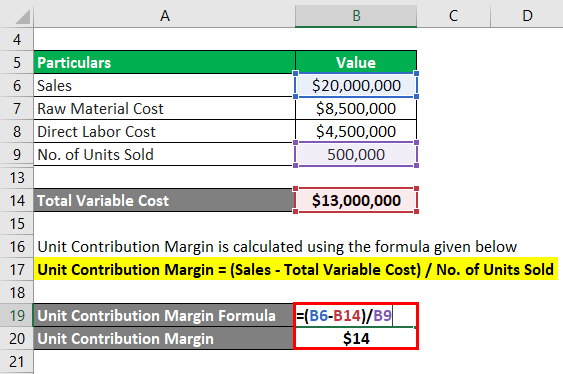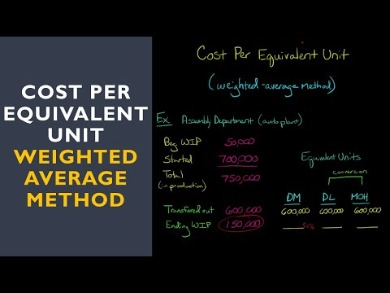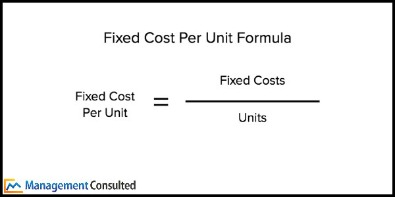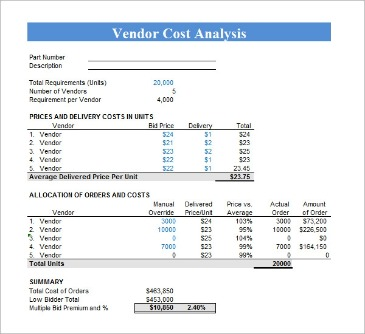Select PageWhen a business is deciding how to find unit cost, they use the unit cost formula. The unit cost formula is used to calculate the cost per unit sold. The formula is the ratio between the total cost and the level of activity in the business. The calculation of the total cost involves the fixed costs and the variable costs. Fixed costs are business expenses that remain constant no matter how much is produced. Examples of fixed costs are machinery or equipment, renting, and insurance. Fixed costs can be forecasted and predictable, which helps businesses with long-term financial planning.

For example, an electricity bill is based on the amount of electricity units consumed in the particular period. Cost driver management increases the efficiency of an organization.Nevertheless, the total fixed cost is also equally important because it is the sum of total fixed cost and total variable cost which when deducted from the revenue will give the company profit. As such, the formula for total cost is very useful for all business. To calculate the total variable costs for a business you have to take into account all the labor and materials needed to produce one unit of a product or service. The total variable cost formula can then be described as the total quantity of output times the variable cost per unit of output. Be careful that you don’t mix up variable cost with variable costing, which is an accounting method used to report variable cost.

## Finops Platforms & Service Providers

This is a prudent choice when the need for increased capacity is not clear. The unit cost is the amount of money it takes to produce one unit of an item. Mathematically, it is the total cost to produce a product divided by the number of units produced.Costs are accumulated by cost centers, whereas these are measured and expressed in terms of cost units. Alternatively, the business may be suffering from a lack of cash so need to sell their products quickly in order to get some cash on hand. It may be to pay for an upcoming debt payment, or, it might just be suffering from illiquidity. At the same time, it might operate a marginal cost pricing strategy to reduce stock – which is particularly common in fashion.

## What If Costs Are Not Entirely Fixed Or Variable?

This way, you can price your goods competitively, and still secure decent sales margins. Monitoring your cost of goods sold helps create context to set pricing and ensure profit is generated. Greg’s Apothecary produces scented candles for an average of \$10 per unit.

• To maximize each unit of production, Coach has branded its products as a luxury item and charges a premium for each unit of production.
• In the case of fixed costs, these are only calculated in marginal cost if these are required to expand production.
• In his second year, he goes on to produce and sell 15 motorbikes for \$150,000, which cost \$75,000 to make.
• It may be to pay for an upcoming debt payment, or, it might just be suffering from illiquidity.
• Factories might choose productive cost centers whereas an administrative wing might choose an unproductive cost center.
• As we can see from the chart below, marginal costs are made up of both fixed and variable costs.
• Fixed costs are independent of your unit production, meaning that no matter how many units you produce or how much demand you have, your fixed cost will stay.

For Greg and many other retail businesses, success is heavily reliant on having a profitable cost per unit — and half of that battle is keeping your costs low. Then the company would take the total cost of \$29,000 and divide by 4000 units.

## Unit Variable Cost

If unit cost, including fixed and variable expenses, is calculated as \$5.00 per unit, then selling a unit for \$6.00 generates a profit of \$1.00 for each sale. A selling price of \$4.00 creates a \$1.00 loss, although this analysis doesn’t accurately capture all market activity. In January, the unit cost of a pen was \$1 if 50,000 units were produced, with direct labor and direct material costs being \$15,000 and \$16,000, respectively. PEN Company LTD wanted to calculate the unit cost of a pen in February with an order totaling 4000 units if direct labor costs and direct material costs are \$6,000 and \$4,000, respectively. High variable cost businesses primarily focus on increasing their pricing power . For each handbag, wallet, etc. that Coach produces, it incurs a variable cost. To maximize each unit of production, Coach has branded its products as a luxury item and charges a premium for each unit of production.So get familiar now with how these costs impact a business, and how a variable-cost-based business model differs from a fixed-cost-based business model. However, the total variable cost can be further expanded into a product of a number of units produced an average variable cost per unit as shown below. Well, the marginal cost looks at the difference between two points https://business-accounting.net/ of production. So how much extra does it cost to produce one unit instead of two units? The change in total cost is therefore calculated by taking away the total cost at point B from the total cost at point A. The cost per unit formula involves the sum of fixed and variable costs, which is then divided by the total number of units manufactured during a period of time.

It is in fact, a primarily variable-cost-based business, which has huge ramifications for how it can and should operate. The final number you need for the cost per unit calculation is the number of units you’re producing. For example, unit cost examples if you are making 100 candles every month, your unit number is 100. This could be the units you produce every month or quarter or you can calculate the cost per unit based on how many units you produce in a given production period.

## Company

If you use 1000 Watts or 1 Kilowatt of power for 1 hour then you consume 1 unit or 1 Kilowatt-Hour of electricity. In the ring Z/nZ of integers modulo n, the units are the congruence classes represented by integers coprime to n. They constitute the multiplicative group of integers modulo n. A unit is an element in a ring that has a multiplicative inverse. If is an algebraic integer which divides every algebraic integer in the field, is called a unit in that field. Connect to a financial advisor in Atlanta, GA if you’d love to learn more about finance.

Impersonal cost center refers to a cost center that consists of a location, item of equipment, or a group of these (e.g., machines, departments, and vehicles). Cost centers can also be divided into operation cost centers and process cost centers, as well as personal cost centers and impersonal cost centers. Given the above, a cost center is, therefore, a natural division of an undertaking that helps to measure and understand operational costs and apply costs to products. Product costs are costs necessary to manufacture a product, while period costs are non-manufacturing costs that are expensed within an accounting period. Long run average cost is the unit cost of producing a certain output when all inputs are variable. Charge cost units to direct costs as they are directly attributed to cost units. While the cost per unit refers to how much you spend to deliver one unit, the price per unit refers to how much you charge customers for each item sold.

For example, if your company creates labels for spice jars, you could have an employee operating the machinery that translates the final design to a special printed paper that attaches to the jar. That employee will increase their productivity over time as they develop their own system for completing their work. Where that employee may start off by producing 100 labels per day, they can grow to produce 200 labels per day.

## How To Calculate Unit Price For Different Units Of Measure

The unit price can be found using a simple formula if the quantity and total cost is known. Simply divide the total price by the quantity to find the unit price. Costs incurred for the purchase of supplies, materials, and fabricated parts to be used or consumed in the process of providing services or products. Examples include chemicals, media, lab supplies, data storage devices, animals, syringes, needles, etc. Each component of a car is a variable cost, including the tires.

• Calculating cost per unit is also important, because it gives ecommerce companies an idea of how much they should charge for each of their products to be profitable.
• Variable costs usually include the cost of labor, material cost, supplies, and energy.
• Instead, sometimes it fluctuates more rapidly, often it fluctuates at a lower rate, and sometimes it fluctuates at the same rate to labor.
• In the second year of business, total costs increase to \$120,000, which include \$85,000 of fixed costs and \$35,000 of variable costs.
• From the company’s overall financial perspective, this may be accurate, but it doesn’t reflect the efficiency of production during the period in which the capital purchase is made.

Calculating a change in quantity involves looking at point A and point B in production and working out the difference. For instance, a business is going to be producing more and more goods as demand increases. However, it is necessary to look at how many more goods are sold between two points in order to calculate how this impacts on final profits.

## The Importance Of Cost Per Unit

While it usually makes little sense to compare variable costs across industries, they can be very meaningful when comparing companies operating in the same industry. They denote the amount of money spent on the production of a product or service and are among the most important analyses a business can run.

If you live outside the area, our financial advisor page will route you to the list of areas we are currently serving. Estimated Cost means estimated cost put to tender for inviting financial bid from the Architect firms/Consultant for Architectural planning, designing and detailing. The Contract Price/Project Cost means the price payable to the Vendor under the Contract for the full and proper performance of its contractual obligations. Indirect cost means any cost not directly identified with a single final cost objective, but identified with two or more final cost objectives or with at least one intermediate cost objective. Please explain why the 2018 Actual Unit Cost Growth \$/Gross Customer Addition calculated by the CEC as \$3,857 is approximately 30% higher than the \$2,976 for 2016 as calculated by the CEC.